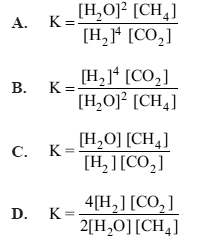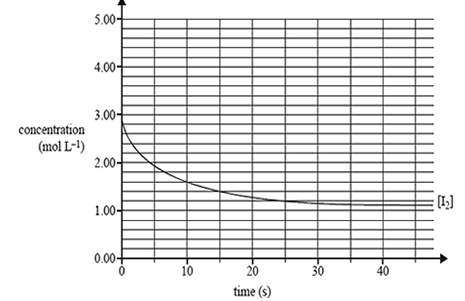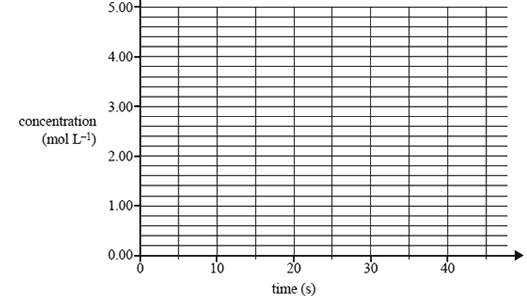Chemical equilibrium (2014 VCE) 1) Hydrogen is produced on an industrial scale from methane. The equation for the reaction is 2H2O(g) + CH4(g) <=> CO2(g) + 4H2(g) The expression for the equilibrium constant for the reverse reaction isSolution2) If an inert gas is added to the equilibrium system, in question 1) above, at a constant temperature and a constant volume, the concentration of hydrogen will A. increase. B. decrease. C. not change. D. decrease then increase. Solution3) A mixture of hydrogen gas and iodine gas is injected into a vessel that is then sealed. The mixture will establish an equilibrium system as described by the following equation. I2(g) + H2(g) <=>2HI(g) In an experiment, 3.00 mol of iodine and 2.00 mol of hydrogen were added to a 1.00 L reaction vessel. The amount of iodine present at equilibrium was 1.07 mol. A constant temperature was maintained in the reaction vessel throughout the experiment. a) Write the expression for the equilibrium constant for this reaction. Solutionb) Determine the equilibrium concentrations of hydrogen and hydrogen iodide, and calculate the value of the equilibrium constant. Solution4) A graph of the decrease in the concentration of I2 until equilibrium is effectively reached is shown below. a) Draw clearly labelled graphs to show how the concentrations of H2 and HI changed over the same period of time.Solutionb) Indicate on the set of axis below how the I2 concentration would have changed if a catalyst had been added to the vessel as well. Assume all other conditions remain the same.Solution